Next: Examples Up: Idea of the algorithm Previous: Idea of the algorithm

### Algorithm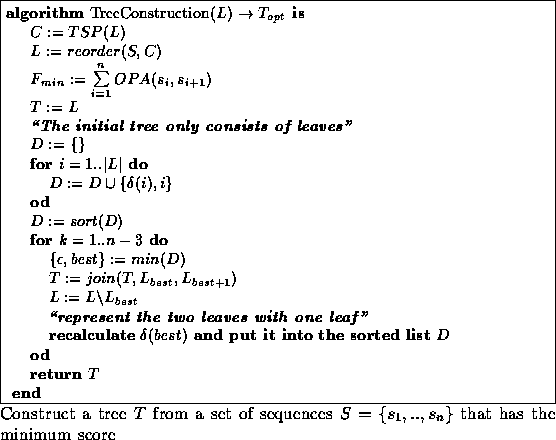Given is a set of sequences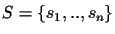. First, the optimal circular order C is calculated with a TSP algorithm. The sequences are renamed with respect to that order. Starting with the optimal circular order C, each of the n-1 pairs of neighboring leaves are swapped (see Algorithm 4.1.1) and the path differenceis calculated for i = 1..n. To save computation time, we initially calculate all n, sort them, and store them in a list D. The best connection is the one with the smallest: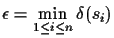. The leaves are connected, and one of the connected leaves is chosen as a representative for the next steps. For the next connection step only two path differences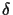have to be recalculated, since nothing else has changed. Since there are n-2 internal nodes (without the root), the total computation needs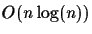for the sorting and linear time in n for the actual tree construction. Once the tree structure is known the exact places of the nodes can be obtained with any least squares method [4,21,12], which takes in the order of n2 time. So given a circular order C, the tree topology can be determined intime. If the edge lengths are to be computed as well, then the computation takes O(n2) time. When only the input sequences are given, then the overall computation time when only input sequences are given is determined by the TSP algorithm.Next: Examples Up: Idea of the algorithm Previous: Idea of the algorithm
Chantal Korostensky
1999-07-14